×

Search anything:

# Merge K sorted Linked Lists

#### Algorithms linked list Sorting AlgorithmsIndian Technical Authorship Contest starts on 1st July 2023. Stay tuned.

We have explained 3 different algorithms to Merge K sorted Linked Lists such that the final Linked List is also sorted.

1. Introduction / Problem Statement
2. Method 1
3. Method 2
4. Method 3
5. Applications

This is similar to Leetcode Problem 23. Merge k Sorted Lists. Let us get started with Merge K sorted Linked Lists.

## Introduction / Problem Statement

In this problem, we are given 'k' number of linked lists. All these lists have already been sorted. We need to merge all the given linked list into a single list and in a sorted order.

Let the first, second and third given linked lists be `1 -> 4 -> 8 -> 10`, `2 -> 3 -> 5` and `6 -> 7 -> 9` respectively. Notice that all of them are already sorted. These lists are represented in the images below.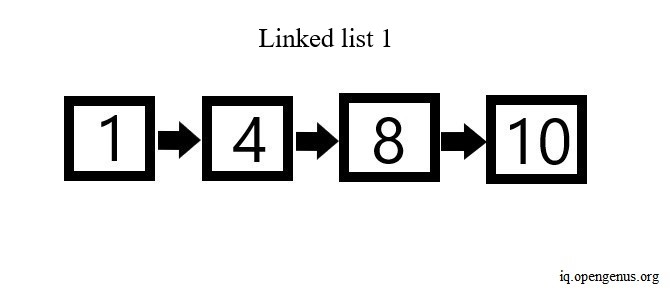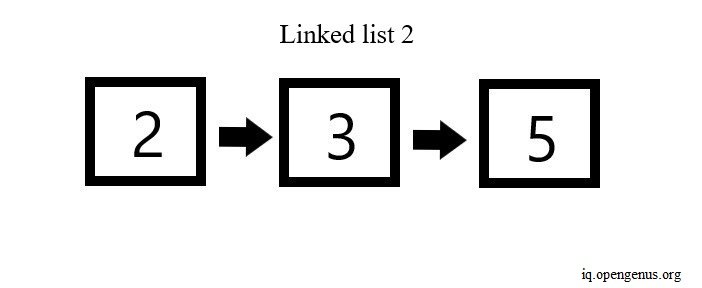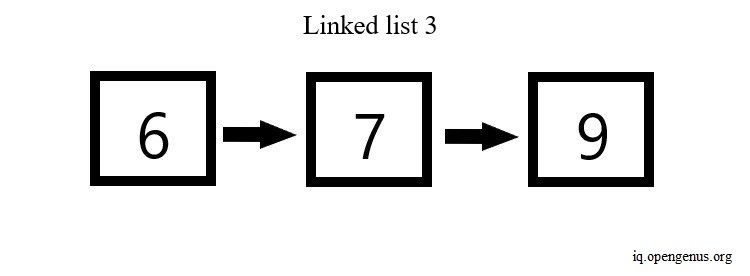So all we need to do now is merge the above linked lists into a single sorted linked list, like the one shown in the image below.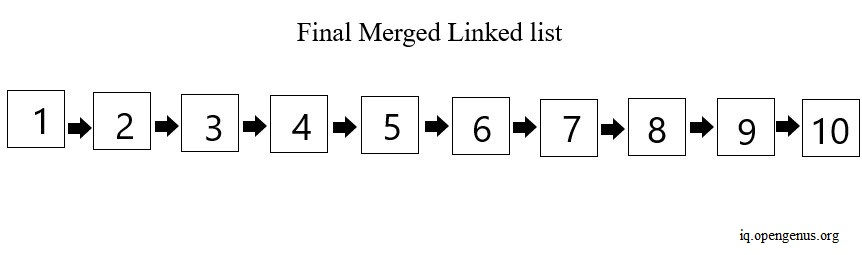There are various approaches to solve this problem. But before we proceed, let's take a quick look at linked lists. Linked lists are a type of data structure, where the values are stored in a list but at different memory locations, instead of being continuous. A linked list is a collection of nodes, and a node is part of the data structure where we can store both a value and reference to the next value (pointer).
Therefore, since a linked list is made up of nodes, and each node is connected to the next node, we need to be careful in our approaches to keep track of each reference/pointer of each node. Otherwise, we might break the list order and lose track of all connected nodes.

Now, let's take a look at some of the approaches we can use to solve this problem.

## Method 1

A simple way to solve this problem is to merge two linked lists at a time and sort them. Within this list, we keep on merging the subsequent linked lists. We do this till all linked lists are merged together into a single sorted linked list. To implement this algorithm, follow the steps mentioned below-

1. Initially, create a function to check all nodes in two lists and use this to merge two lists into a single one. To do this, keep track of the current first nodes and pointers of both lists using two variables. Then using a while loop keep checking the values from both lists till we reach a point where the value becomes `None`. If this happens, it implies that we have reached the end of the list and we break out of the loop. Otherwise, compare the values and set the pointers accordingly. This way, we create a helper function that we use in the next step.

2. Now, create a function, where we check the first nodes of each lists and sort them using the function we created in step one.

3. In the end, we finally create a function that takes a list containing the first nodes of every linked list, and output a single sorted merged list. We first merge two lists into a single one and into this, we merge the next linked list, one by one.

4. Using a helper print function, we print the node values of the final merged list.

Implementation example

The following code executes the algorithm discussed above.

``````# Python program to merge k sorted linked lists in-place

# Create a class called 'Node' with one part to store value
# and other part to store pointer/reference to next value
class Node:
def __init__(self, data):
self.data = data
self.next = None

# A function to print all values in a linked list

while (node != None) :
print( node.data, end =" ")
node = node.next

# Merges two lists
# lst 1 and lst 2 are the first node in each list respectively
# this function changes the pointers in  a sorted order
# so all values get arranged in order
# we finally merge all values together in the next function
def merge_twolists(lst1, lst2):

# if only one node in first list
# make first list's node point to second list's first node
if (lst1.next == None) :
lst1.next = lst2
return lst1

# keep track of current first nodes
# and next pointers of both lists

curr1 = lst1
next1 = lst1.next
curr2 = lst2
next2 = lst2.next

while (next1 != None and curr2 != None):

# if the first node of list 2
# contains value greater than first node of list 1
# but is smaller than second node of list 1
# change pointers accordingly
if ((curr2.data) >= (curr1.data) and
(curr2.data) <= (next1.data)) :
next2 = curr2.next
curr1.next = curr2
curr2.next = next1

# set curr1 and curr2
# point to their immediate next pointers
curr1 = curr2
curr2 = next2

else :
# if values are already in order and
# more nodes exist in first list
# shift curr 1 to point to next value in list 1
if (next1.next) :
next1 = next1.next
curr1 = curr1.next

# if values are already in order and
# no nodes further exist in list 1
# make the last node of first list point to
# second list's remaining nodes
else :
next1.next = curr2
return lst1

return lst1

# Compare first nodes in each list
# and call merge_twolists() to merge lists
# Lists are are finally merged in place
def merge(lst1, lst2):

if (lst1 == None):
return lst2
if (lst2 == None):
return lst1

# first use the linked list
# whose first node's data is the smallest
if (lst1.data < lst2.data):
return merge_twolists(lst1, lst2)
else:
return merge_twolists(lst2, lst1)

# function to merge all lists into a single linked list
# two lists are merged together at a time
# n is the number of linked lists to be merged
# lst is the main list containing all linked lists to merge
def  mergeKlists (lst,n):
if n == 1:
return lst
if n == 0:
return None
# first merge first two linked lists
mergedlist = merge(lst,lst)

# into the linked list created in the previous step
for i in range(2,n):
mergedlist = merge(mergedlist,lst[i])
return mergedlist

# example to test above programs
# create a total of 4 linked list
k = 4
mlist = [i for i in range(k)]

mlist = Node(0)
mlist.next = Node(2)
mlist.next.next = Node(4)

mlist = Node(1)
mlist.next = Node(3)
mlist.next.next = Node(5)

mlist = Node(6)
mlist.next = Node(7)
mlist.next.next = Node(9)

mlist = Node(8)
mlist.next = Node(10)

mergedlists = mergeKlists(mlist, k)

``````

The above program produces the following output-

``````0 1 2 3 4 5 6 7 8 9 10
``````

Complexity

• Time: in the worst case, the runtime required comes out to be O(n(k^2)), where n is the number of nodes in each linked list, and k is the number of linked lists. This because at each step we go through all nodes in the respective linked lists being merged and we repeat this step till all linked lists are merged together.
• Space: the space requirement turns out to be O(N) in the worst case, where N is the total number of nodes combining all linked lists.

## Method 2

Another way to solve this problem is using the min-heap data structure. A min-heap is a binary tree where every node's value is greater than or equal to the root/parent node's value. The parent/root node contains the smallest/minimum value. This is depicted in the diagram below.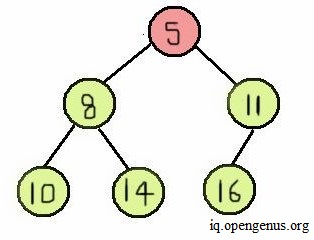To solve this problem using min-heap, follow the steps below-

1. When creating the class `Node`, modify the `__lt()__` special method within the class structure. We do this because this method allows us to call and use the less than (`<`) operator. Since we are implementing min-heap in our solution, we need to check for minimum values. This way, we can do so and work with min-heap using our `Node` structure.

2. After importing the `heapq` module and `heappop`,`heappush` methods, we directly create a function to merge all linked lists into a single one. For this, we first a list (`m_heap`) that contains first node of each linked list and convert this list into a heap structure. Then we create two variables to keep track of the first and last node of the final output list. Initially, we set them equal to `None`.

3. Then using a while loop we look through all elements in our heap structure (`m_heap`). We get the smallest value from the heap using `heappop` method and set this value as the first and the last node included in the final output list, in case the first node does not contain any value. Otherwise, we set only the last node to point to this value.

4. After that, we check if the minimum value that we included in the final list also has a pointer to another value, from the same list we took the smallest value. If the pointer is not `None`, we insert this value to the heap. We keep doing this till we empty the heap. In the end, we return the first head node of the list.

5. Using a helper function, we print all the node values in the final merged list we get from step 4.

Implementation example

The code below implements the algorithm discussed above.

``````# import heapq module to use the heap data structure
# in a min-heap structure  every node' value is greater than
# or equal to the parent node's value
# therefore the parent/root node contains the smallest/minimum value
import heapq
from heapq import heappop, heappush

class Node:
def __init__(self, data):
self.data = data
self.next = None

# we update the `__lt__()` special method within the `Node` class
def __lt__(self, other):
return self.data < other.data

# a function to print values of a linked list
def print_list(node):
while node:
print(node.data, end=' ')
node = node.next

# We use this function to merge all `k` sorted linked lists.
# As input, this function takes a list of lists
# and then returns the final sorted merged list
def mergeKLists(lists):

# using the first node of every list
# we create a min-heap structure
m_heap = [i for i in lists]
heapq.heapify(m_heap)

# create two pointer variables
# variable 'first' points to first node of the final output list
# 'last' variable points to the last node of the final output list
first = last = None

# loop through all elements in min-heap till it gets empty
while m_heap:

# get the smallest valued node from the min-heap
min = heappop(m_heap)

# put the smallest value node in the final output list
if first is None:
first = min
last = min
else:
last.next = min
last = min

# move to the next node
# from the same list we took the smallest valued node
# insert it into min-heap
if min.next:
heappush(m_heap, min.next)

# return the first node of the final merged list
return first

# an EXAMPLE to test above program

# total number of linked lists
k = 3

# a list to store the first nodes of all linked lists
mlists = [i for i in range(k)]

mlists = Node(1)
mlists.next = Node(5)
mlists.next.next = Node(7)

mlists = Node(2)
mlists.next = Node(3)
mlists.next.next = Node(6)
mlists.next.next.next = Node(9)

mlists = Node(4)
mlists.next = Node(8)
mlists.next.next = Node(10)

# Merge all lists into a single merged sorted list
# Print final merged list
final_list = mergeKLists(mlists)
print_list(final_list)

``````

The above program produces the following output.

``````1 2 3 4 5 6 7 8 9 10
``````

Complexity

1. Time: this algorithm has a total runtime of O(nk(log(k)) in the worst case, where k is the number of linked lists and n is the number of nodes in each linked list. This is because insertion and deletion in heap structure takes O(log(k)) runtime and we do this for all nodes of every linked list till all lists are combined.

2. Space: in the best case, the space requirement would be O(1), and in the worst case the requirement would be O(k), where k represents the number of linked lists.

## Method 3

We can solve also solve this problem using an intuition we applied in merge sort. We can merge all lists by pairing up two lists and then merge them. Let the total number of linked lsits be 'k'. In the initial iteration, we'd have k/2 number of lists left, and in the next iteration we'd have k/4 number of lists left, and this will continue till we merge all linked lists into a single one and only one list we'd be left. To implement this algorithm, we perform the following steps-

1. We initially create a function to sort and merge two lists into a single one. We check if the first node of each list is `None` and if so, we return the other list. Then we check if the first node of list 1 is less than or equal to that of list 2. We can do this either for list 1 or list 2. Then we set the first node of the final list (`result` variable) equal to the first node of list 1 and recursively call the same function to get the pointer variable. We do this till we reach the end of both lists and the pointer variable becomes `None`. In the end, we return the first node of the final list.

2. We then create a separate function to merge all linked lists into a single one. This function takes in a list of first nodes from each linked list. Parameter 'k' represents the number of linked lists.

3. Using a variable called `last`, we keep track of the number of linked lists. Then using a while loop, we go through all linked lists till the `last` variable becomes one. We create two variables `i` and `j` to keep track of each pair of linked lists. We set `i` equal to zero and `j` equal to the `last` variable. Then using another while loop, we merge all linked lists till both `i` and `j` are unequal and `i` stays greater than `j`. Otherwise, it'd imply an overlap of pairs of linked lists, and we'd merge the wrong lists. We keep on incrementing `i` and decrese `j`. If `i` becomes greater than or equal to `j`, we set the `last` variable equal to j. This way, in the end when `j` becomes zero, we break out of the loop. We then return the first list into which we merged all the linked lists.

4. Using a helper function, we print all the node values in the final merged list we get from the previous step.

Implementation example

The following program executes the algorithm explained above.

``````# A program to merge k sorted lists

# create a Node class
class Node:

def __init__(self,data):

self.data = data
self.next = None

# Function to print all node values in a linked list
def printlist(node):

while (node != None):
print(node.data, end = ' ')
node = node.next

# a function to create a new sorted linked list
# this function takes in two linked lists to create a new merged one
# lst1 and lst2 are the first head node of the two lists respectively
def Sort_Merge(lst1, lst2):

# Base cases
if (lst1 == None):
return(lst2)
elif (lst2 == None):
return(lst1)

# Choose either lst1 or lst2
# we use recursion to get the smallest values in sorted order
if (lst1.data <= lst2.data):
result = lst1
result.next = Sort_Merge(lst1.next, lst2)
else:
result = lst2
result.next = Sort_Merge(lst1, lst2.next)
return result

# a function to create a single sorted linked list
# combining all linked lists into a single one
def mergeKLists(lst, k):

# merge and sort til only one single list exists
last = k-1
while (last != 0):
i = 0
j = last

# i and j must be unequal
# otherwise we'd overlap lists when merging and sorting
# leading to faulty final merged list output
while (i < j):

# merge two lists at a time
# and assign the merged list into a single list
lst[i] = Sort_Merge(lst[i], lst[j])

# increment i and decrease j
# this way to merge and sort the correct pairs of lists
i += 1
j -= 1
# In case all correct pairs are merged,
# we update last variable
# i >= j implies overlap of already merged lists
# and we should stop before that happens
# setting last = j would stop the while loop
# as in the end j would become less than i or 0
# so we would break out of the loop
if (i >= j):
last = j

# in the end, all lists get merged in the first linked list
# that's why we return lst
return lst

# an example to test the above program

# k denotes the number of linked lists
k = 3

# a list to store the first nodes of all linked lists
mlist = [i for i in range(k)]

mlist = Node(1)
mlist.next = Node(3)
mlist.next.next = Node(5)
mlist.next.next.next = Node(7)

mlist = Node(2)
mlist.next = Node(4)
mlist.next.next = Node(6)
mlist.next.next.next = Node(8)

mlist = Node(0)
mlist.next = Node(9)
mlist.next.next = Node(10)
mlist.next.next.next = Node(11)

# call mergeKLists to merge all lists into a single sorted one
final_list = mergeKLists(mlist, k)

# print final merged linked list
printlist(final_list)
``````

The above program produces the following output.

``````0 1 2 3 4 5 6 7 8 9 10 11
``````

Complexity

• Time: The while loop we use to merge linked lists into a single one takes a runtime of O(log(k)), where k is the number of linked lists. We use the while loop to go through all nodes in each linked list till every linked list is merged into the final linked list, so the total runtime and complexity turns out to be O(nk(log(k)) in the worst case, where n is the number of nodes in each linked list.
• Space: the space requirement for this program turns out to be O(N) in the worst case (because we use recursion for every node), where N is the total number of nodes combining all linked lists.

## Applications

• Linked lists are fundamentally used to store data in an organized manner, such as in the form of stacks and queues, where the data is stored in an order and can be easily edited/added later on. This is a vital part of computer programming and computer organisation.

• In general, linked lists help keep track of data as a collection and tell how parts of data are connected with each other. Therefore, they are used in a variety of applications such as movie players, music players, browsers, user interfaces, image viewers, calendar systems, games etc.

With this article at OpenGenus, you must have the complete idea of how to merge K sorted Linked Lists.